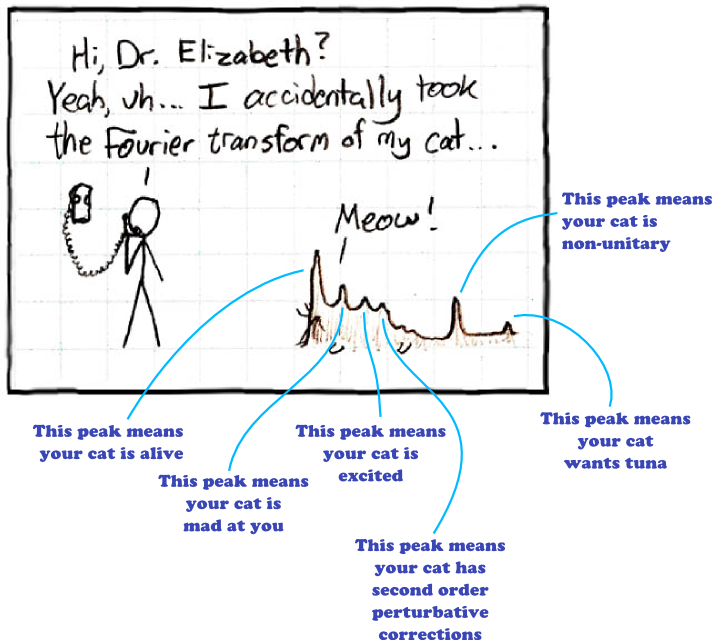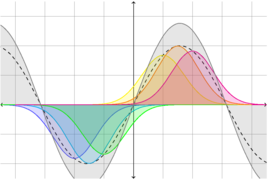1. 2013
Sep
24

## Interpreting Fourier transformsBefore you ask: yes, I do know I’ve been absent from blogging for too long. About a month, actually, which roughly coincides with the start of the fall semester here at Penn State. I’m TAing a class this semester, and also working overtime to push out a paper about my group’s current research (a followup to this one, which I will be writing about soon), and still moderating Physics Stack Exchange, and many other things which just haven’t left time for blogging as much as I would have liked.

There are some recent nifty bits of physics I do plan to write about, but until I get time to do that, enjoy this picture:

(adapted from XKCD). I was inspired by last week’s physics colloquium speaker, Patrick Drew, who actually works on neural engineering. Not very physicsy, you’d think, but actually physics has ties to all sorts of other fields of study because in the end we’re all just trying to make models that describe reality.

2. 2010
Sep
28

## Convolution and the Fourier transformOne of the neat properties of the Fourier transform is that if you want to convolve two functions, an easy way to do it is to multiply their Fourier transforms together and then take the inverse Fourier transform of the result. It’s often said as “convolution in normal space corresponds to multiplication in Fourier space.” I think I’ve always accepted this on faith, but today I had occasion to actually come up with a proof.

To start with, let’s make some definitions. The convolution of two functions $$f(x)$$ and $$g(x)$$ is the result of shifting and scaling one function by each point of the other. It’s usually used in applications where one of the functions is a Gaussian distribution, or some other function that has a peak at some point, and in that case you can think of it like this: suppose $$g(x)$$ is the function with the peak. For each point $$\bigl(y,f(y)\bigr)$$, make a copy of $$g(x)$$, and shift it over so that the peak is at $$y$$. Then multiply it by the value of $$f(y)$$. The sum of all those scaled and shifted peaks is …